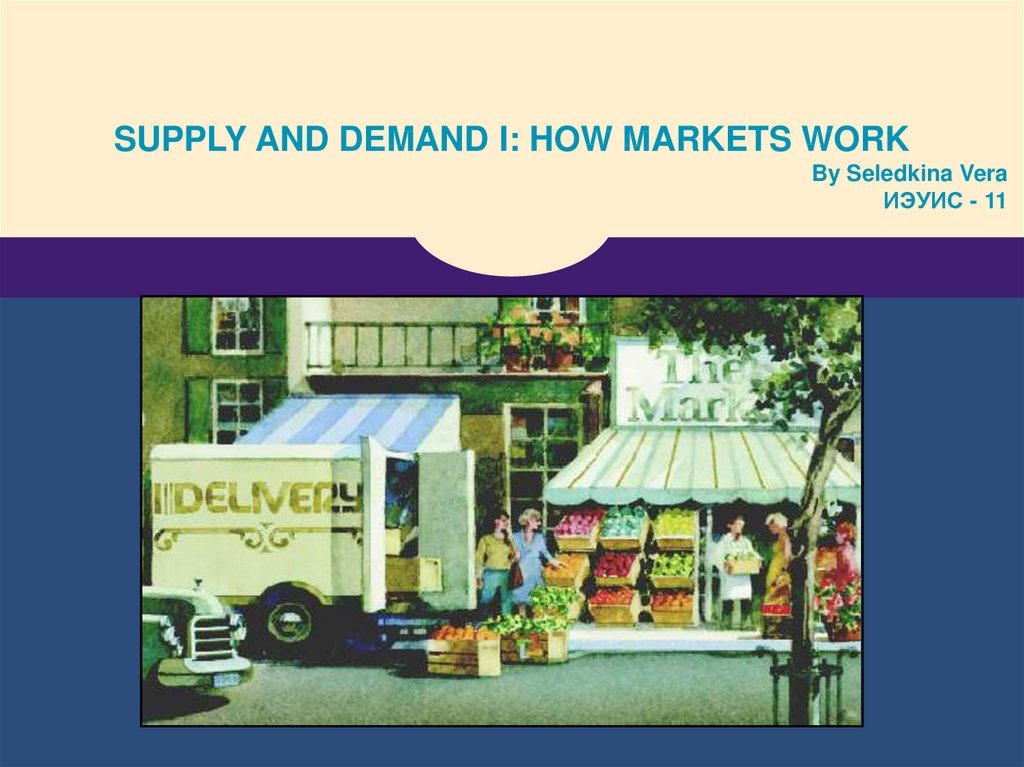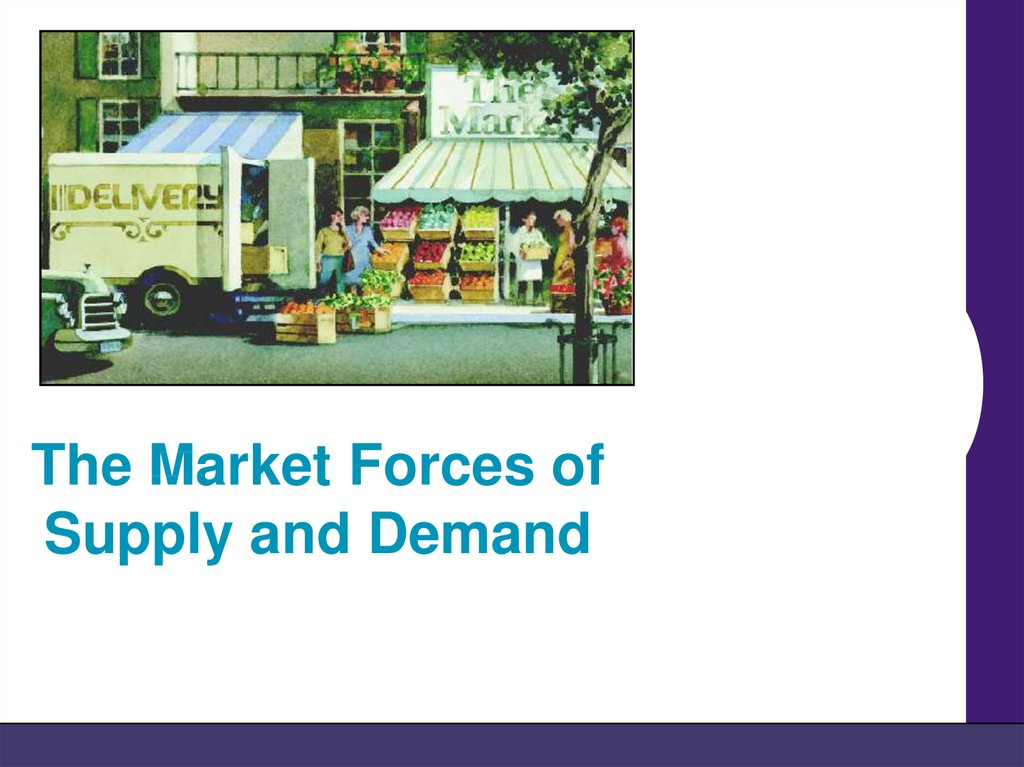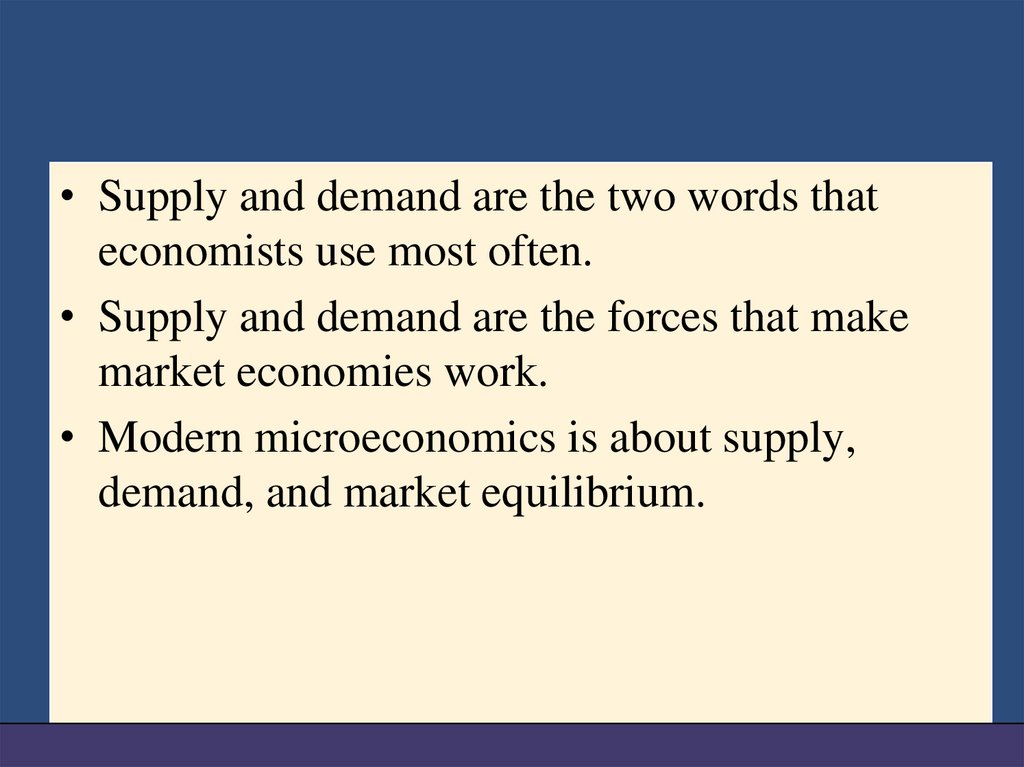# The market forces of supply and demand

## 1.

SUPPLY AND DEMAND I: HOW MARKETS WORK
By Seledkina Vera
ИЭУИС - 11

## 2.

The Market Forces of
Supply and Demand

## 3.

• Supply and demand are the two words that
economists use most often.
• Supply and demand are the forces that make
market economies work.
• Modern microeconomics is about supply,
demand, and market equilibrium.

## 4. MARKETS AND COMPETITION

• A market is a group of buyers and sellers of a
particular good or service.
• The terms supply and demand refer to the
behavior of people . . . as they interact with one
another in markets.

## 5. MARKETS AND COMPETITION

• Sellers determine supply

## 6. Competitive Markets

• A competitive market is a market in which there
are many buyers and sellers so that each has a
negligible impact on the market price.

## 7. Competition: Perfect and Otherwise

• Perfect Competition
• Products are the same
• Numerous buyers and sellers so that each has no
influence over price
• Buyers and Sellers are price takers
• Monopoly
• One seller, and seller controls price

## 8. Competition: Perfect and Otherwise

• Oligopoly
• Few sellers
• Not always aggressive competition
• Monopolistic Competition
• Many sellers
• Slightly differentiated products
• Each seller may set price for its own product

## 9. DEMAND

• Quantity demanded is the amount of a good that
buyers are willing and able to purchase.
• Law of Demand
• The law of demand states that, other things equal,
the quantity demanded of a good falls when the
price of the good rises.

## 10. The Demand Curve: The Relationship between Price and Quantity Demanded

• Demand Schedule
• The demand schedule is a table that shows the
relationship between the price of the good and the
quantity demanded.

## 12. The Demand Curve: The Relationship between Price and Quantity Demanded

• Demand Curve
• The demand curve is a graph of the relationship
between the price of a good and the quantity
demanded.

## 13. Figure 1 Catherine’s Demand Schedule and Demand Curve

Price of
Ice-Cream Cone
\$3.00
2.50
1. A decrease
in price ...
2.00
1.50
1.00
0.50
0 1 2 3 4 5 6 7 8 9 10 11 12 Quantity of
Ice-Cream Cones
2. ... increases quantity
of cones demanded.

## 14. Market Demand versus Individual Demand

• Market demand refers to the sum of all
individual demands for a particular good or
service.
• Graphically, individual demand curves are
summed horizontally to obtain the market
demand curve.

## 15. Shifts in the Demand Curve

• Change in Quantity Demanded
• Movement along the demand curve.
• Caused by a change in the price of the product.

## 16. Changes in Quantity Demanded

Price of IceCream
Cones
B
\$2.00
A tax that raises the
price of ice-cream
cones results in a
movement along the
demand curve.
A
1.00
D
0
4
8
Quantity of Ice-Cream Cones

## 17. Shifts in the Demand Curve

Consumer income
Prices of related goods
Tastes
Expectations

## 18. Shifts in the Demand Curve

• Change in Demand
• A shift in the demand curve, either to the left or
right.
• Caused by any change that alters the quantity
demanded at every price.

## 19. Shifts in the Demand Curve

• Consumer Income
• As income increases the demand for a normal good
will increase.
• As income increases the demand for an inferior
good will decrease.

## 20. Shifts in the Demand Curve

• Prices of Related Goods
• When a fall in the price of one good reduces the
demand for another good, the two goods are called
substitutes.
• When a fall in the price of one good increases the
demand for another good, the two goods are called
complements.

## 21. SUPPLY

• Quantity supplied is the amount of a good that
sellers are willing and able to sell.
• Law of Supply
• The law of supply states that, other things equal, the
quantity supplied of a good rises when the price of
the good rises.

## 22. The Supply Curve: The Relationship between Price and Quantity Supplied

• Supply Schedule
• The supply schedule is a table that shows the
relationship between the price of the good and the
quantity supplied.

## 23. The Supply Curve: The Relationship between Price and Quantity Supplied

• Supply Curve
• The supply curve is the graph of the relationship
between the price of a good and the quantity
supplied.

## 24. Market Supply versus Individual Supply

• Market supply refers to the sum of all
individual supplies for all sellers of a particular
good or service.
• Graphically, individual supply curves are
summed horizontally to obtain the market
supply curve.

## 25. Shifts in the Supply Curve

Input prices
Technology
Expectations
Number of sellers

## 26. Shifts in the Supply Curve

• Change in Quantity Supplied
• Movement along the supply curve.
• Caused by a change in anything that alters the
quantity supplied at each price.

## 27. Shifts in the Supply Curve

• Change in Supply
• A shift in the supply curve, either to the left or right.
• Caused by a change in a determinant other than
price.

## 28. SUPPLY AND DEMAND TOGETHER

• Equilibrium refers to a situation in which the
price has reached the level where quantity
supplied equals quantity demanded.

## 29. SUPPLY AND DEMAND TOGETHER

• Equilibrium Price
• The price that balances quantity supplied and
quantity demanded.
• On a graph, it is the price at which the supply and
demand curves intersect.
• Equilibrium Quantity
• The quantity supplied and the quantity demanded at
the equilibrium price.
• On a graph it is the quantity at which the supply and
demand curves intersect.

## 30. Equilibrium

• Surplus
• When price > equilibrium price, then quantity
supplied > quantity demanded.
• There is excess supply or a surplus.
• Suppliers will lower the price to increase sales, thereby
moving toward equilibrium.

## 31. Equilibrium

• Shortage
• When price < equilibrium price, then quantity
demanded > the quantity supplied.
• There is excess demand or a shortage.
• Suppliers will raise the price due to too many buyers
chasing too few goods, thereby moving toward
equilibrium.